# The Dual

[Page A-30 ( continued )]

Every linear programming model has two forms: the primal and the dual . The original form of a linear programming model is called the primal. All the examples in this module are primal models. The dual is an alternative model form derived completely from the primal. The dual is useful because it provides the decision maker with an alternative way of looking at a problem. Whereas the primal gives solution results in terms of the amount of profit gained from producing products, the dual provides information on the value of the constrained resources in achieving that profit.

The original linear programming model is called the primal , and the alternative form is the dual .

The following example will demonstrate how the dual form of a model is derived and what it means. The Hickory Furniture Company produces tables and chairs on a daily basis. Each table produced results in \$160 in profit; each chair results in \$200 in profit. The production of tables and chairs is dependent on the availability of limited resources labor, wood, and storage space. The resource requirements for the production of tables and chairs and the total resources available are as follows .

The dual solution variables provide the value of the resources, that is, shadow prices .

Resource Requirements

Resource

T ABLE

C HAIR

Total Available per Day

Labor (hr)

2

4

40

Wood (bd ft)

18

18

216

Storage (ft 2 )

24

12

240

The company wants to know the number of tables and chairs to produce per day to maximize profit. The model for this problem is formulated as follows.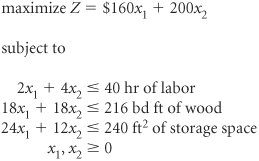[Page A-31]

where

x 1 = number of tables produced

x 2 = number of chairs produced

This model represents the primal form. For a primal maximization model, the dual form is a minimization model. The dual form of this example model is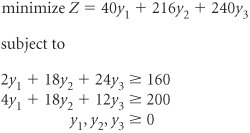The dual is formulated entirely from the primal.

The specific relationships between the primal and the dual demonstrated in this example are as follows.

1. The dual variables, y 1 , y 2 , and y 3 , correspond to the model constraints in the primal. For every constraint in the primal there will be a variable in the dual. For example, in this case the primal has three constraints ; therefore, the dual has three decision variables .

2. The quantity values on the right-hand side of the primal inequality constraints are the objective function coefficients in the dual. The constraint quantity values in the primal, 40, 216, and 240, form the dual objective function: Z = 40 y 1 + 216 y 2 + 240 y 3 .

3. The model constraint coefficients in the primal are the decision variable coefficients in the dual. For example, the labor constraint in the primal has the coefficients 2 and 4. These values are the y 1 variable coefficients in the model constraints of the dual: 2 y 1 and 4 y 1 .

4. The objective function coefficients in the primal, 160 and 200, represent the model constraint requirements (quantity values on the right-hand side of the constraint) in the dual.

5. Whereas the maximization primal model has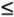constraints, the minimization dual model has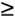constraints.

The primaldual relationships can be observed by comparing the two model forms shown in Figure A-9.

##### (This item is displayed on page A-32 in the print version)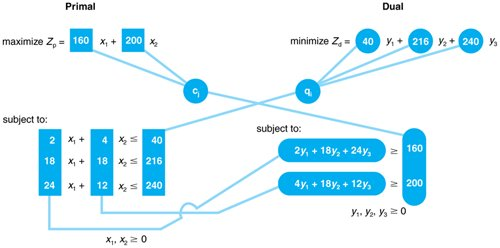Now that we have developed the dual form of the model, the next step is determining what the dual means. In other words, what do the decision variables y 1 , y 2 , and y 3 mean, what do themodel constraints mean, and what is being minimized in the dual objective function?

A primal maximization model withconstraints converts to a dual minimization model withconstraints, and vice versa.

#### Interpreting the Dual Model

The dual model can be interpreted by observing the simplex solution to the primal form of the model. The simplex solution to the primal model is shown in Table A-32.

##### (This item is displayed on page A-32 in the print version)

Basic Variables

Quantity

160

200

c j

x 1

x 2

s 1

s 2

s 3

200

x 2

8

1

1/2

1/18

160

x 1

4

1

1/2

1/9

s 3

48

6

2

1

z j

2,240

160

200

20

20/3

c j z j

20

20/3

Interpreting this primal solution, we have

 x 1 = 4 tables x 2 = 8 chairs s 3 = 48 ft 2 of storage space Z = \$2,240 profit

[Page A-32]

This optimal primal tableau also contains information about the dual. In the c j z j row of Table A-32, the negative values of 20 and 20/3 under the s 1 and s 2 columns indicate that if one unit of either s 1 or s 2 were entered into the solution, profit would decrease by \$20 or \$6.67 (i.e., 20/3), respectively.

Recall that s 1 represents unused labor and s 2 represents unused wood. In the present solution s 1 and s 2 are not basic variables, so they both equal zero. This means that all of the material and labor are being used to make tables and chairs, and there are no excess ( slack ) labor hours or board feet of material left over. Thus, if we enter s 1 or s 2 into the solution, then s 1 or s 2 no longer equals zero, we would be decreasing the use of labor or wood. If, for example, one unit of s 1 is entered into the solution, then one unit of labor previously used is not used, and profit is reduced by \$20.

Let us assume that one unit of s 1 has been entered into the solution so that we have one hour of unused labor ( s 1 = 1). Now let us remove this unused hour of labor from the solution so that all labor is again being used. We previously noted that profit was decreased by \$20 by entering one hour of unused labor; thus, it can be expected that if we take this hour back (and use it again), profit will be increased by \$20. This is analogous to saying that if we could get one more hour of labor, we could increase profit by \$20. Therefore, if we could purchase one hour of labor, we would be willing to pay up to \$20 for it because that is the amount by which it would increase profit.

[Page A-33]

## Time Out: For John Von Neumann

John Von Neumann, the famous Hungarian mathematician , is credited with many contributions in science and mathematics, including crucial work on the development of the atomic bomb during World War II and the development of the computer following the war. In 1947 George Dantzig visited Von Neumann at the Institute for Advanced Study at Princeton and described the linear programming technique to him. Von Neumann grasped the technique immediately, because he had been working on similar concepts himself, and went on to explain duality to Dantzig for the first time.

The negative c j z j row values of \$20 and \$6.67 are the marginal values of labor ( s 1 ) and wood ( s 2 ), respectively. These dual values are also often referred to as shadow prices , since they reflect the maximum "price" one would be willing to pay to obtain one more unit of the resource.

The c j z j values for slack variables are the marginal values of the constraint resources, i.e., shadow prices.

What happened to the third resource, storage space? The answer can be seen in Table A-32. Notice that the c j z j row value for s 3 (which represents unused storage space) is zero. This means that storage space has a marginal value of zero; that is, we would not be willing to pay anything for an extra foot of storage space.

If a resource is not completely used, i.e., there is slack, its marginal value is zero.

The reason more storage space has no marginal value is because storage space was not a limitation in the production of tables and chairs. Table A-32 shows that 48 square feet of storage space were left unused (i.e., s 3 = 48) after the 4 tables and 8 chairs were produced. Since the company already has 48 square feet of storage space left over, an extra square foot would have no additional value; the company cannot even use all of the storage space it has available.

We need to consider one additional aspect of these marginal values. In our discussion of the marginal value of these resources, we have indicated that the marginal value (or shadow price ) is the maximum amount that would be paid for additional resources. The marginal value of \$60 for one hour of labor is not necessarily what the Hickory Furniture Company would pay for an hour of labor. This depends on how the objective function is defined. In this example we are assuming that all of the resources available, 40 hours of labor, 216 board feet of wood, and 240 square feet of storage space, are already paid for. Even if the company does not use all the resources, it still must pay for them. They are sunk costs. In other words, the cost of any additional resources secured are included in the objective function coefficients. As such, the profit values in the objective function for each product are unaffected by how much of a resource is actually used; the profit is independent of the resources used. If the cost of the resources is not included in the profit function, then securing additional resources would reduce the marginal value.

The shadow price is the maximum amount that should be paid for one additional unit of a resource.

The shadow price is the maximum amount that should be paid for one additional unit of a resource.

Continuing our analysis, we note that the profit in the primal model was shown to be \$2,240. For the furniture company, the value of the resources used to produce tables and chairs must be in terms of this profit. In other words, the value of the labor and wood resources is determined by their contribution toward the \$2,240 profit. Thus, if the company wanted to assign a value to the resources it used, it could not assign an amount greater than the profit earned by the resources. Conversely, using the same logic, the total value of the resources must also be at least as much as the profit they earn. Thus, the value of all the resources must exactly equal the profit earned by the optimal solution.

The total marginal value of the resources equals the optimal profit.

Now let us look again at the dual form of the model.

[Page A-34]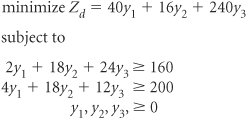The dual variables equal the marginal value of the resources, the shadow prices.

Given the previous discussion on the value of the model resources, we can now define the decision variables of the dual, y 1 , y 2 , and y 3 , to represent the marginal values of the resources:

y 1 = marginal value of 1 hr of labor = \$20

y 2 = marginal value of 1 bd ft of wood = \$6.67

y 3 = marginal value of 1 ft 2 of storage space = \$0

#### Use of the Dual

The importance of the dual to the decision maker lies in the information it provides about the model resources. Often the manager is less concerned about profit than about the use of resources because the manager often has more control over the use of resources than over the accumulation of profits. The dual solution informs the manager of the value of the resources, which is important in deciding whether or not to secure more resources and how much to pay for these additional resources.

The dual provides the decision maker with a basis for deciding how much to pay for more resources.

If the manager secures more resources, the next question is "How does this affect the original solution?" The feasible solution area is determined by the values forming the model constraints, and if those values are changed, it is possible for the feasible solution area to change. The effect on the solution of changes to the model is the subject of sensitivity analysis, the next topic to be presented here.Introduction to Management Science (10th Edition)
ISBN: 0136064361
EAN: 2147483647
Year: 2006
Pages: 358

Similar book on Amazon

flylib.com © 2008-2017.
If you may any questions please contact us: flylib@qtcs.net